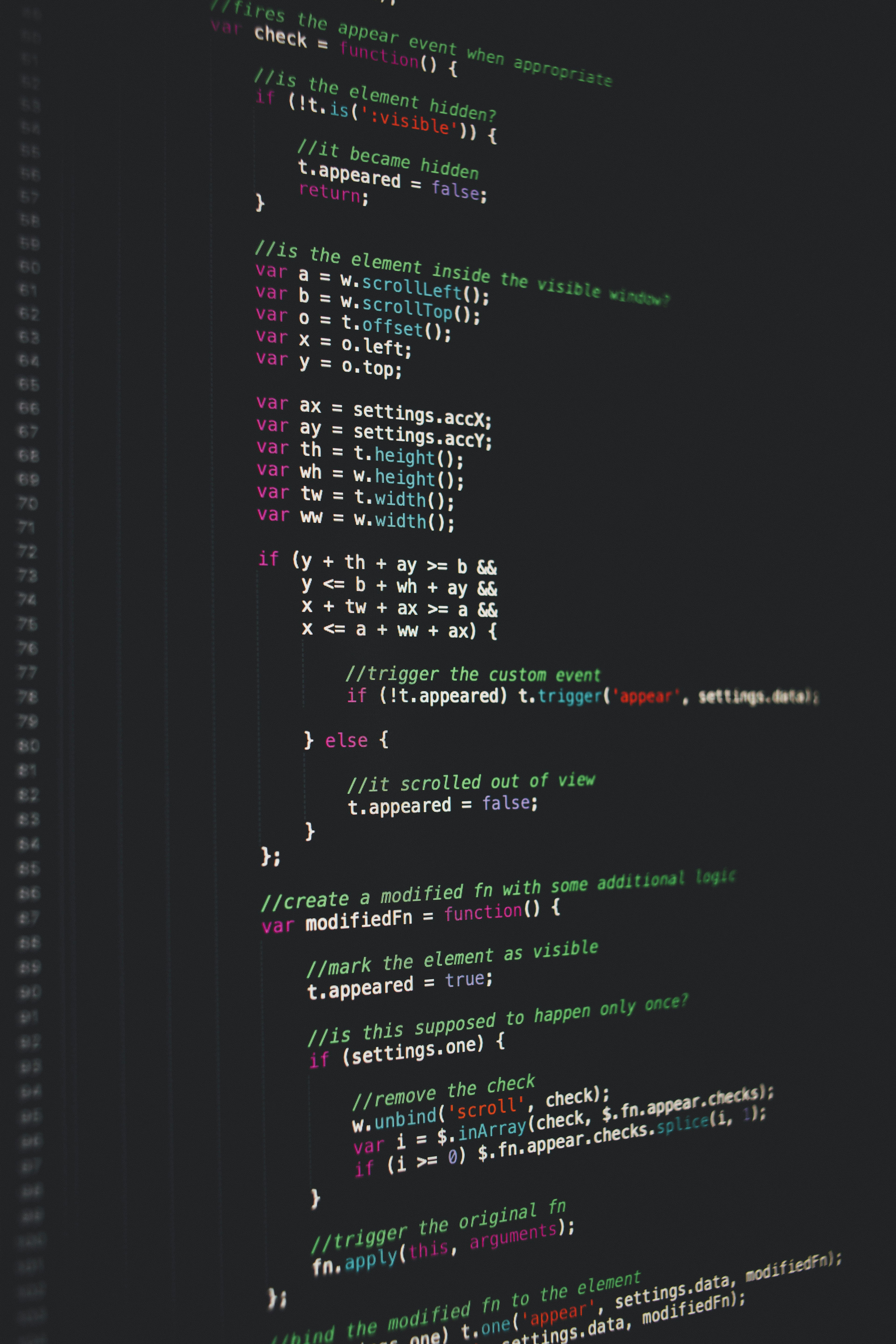Hackerrank Strings Solution

# Hackerrank Strings SolutionC++ provides a nice alternative data type to manipulate strings, and the data type is conveniently called string. Some of its widely used features are the following:

Declaration:

string a = "abc";


Size:

int len = a.size();


Concatenate two strings:

string a = "abc";
string b = "def";
string c = a + b; // c = "abcdef".


Accessing  element:

string s = "abc";
char   c0 = s;   // c0 = 'a'
char   c1 = s;   // c1 = 'b'
char   c2 = s;   // c2 = 'c'

s = 'z';         // s = "zbc"


P.S.: We will use cin/cout to read/write a string.

Input Format

You are given two strings,  and , separated by a new line. Each string will consist of lower case Latin characters ('a'-'z').

Output Format

In the first line print two space-separated integers, representing the length of  and  respectively.
In the second line print the string produced by concatenating  and  ().
In the third line print two strings separated by a space,  and .  and  are the same as  and , respectively, except that their first characters are swapped.

Sample Input

abcd
ef


Sample Output

4 2
abcdef
ebcd af


Explanation

• "abcd"
• "ef"
• "abcdef"
• "ebcd"
• "af"

### Solution in cpp

Approach 1.

#include <iostream>
#include <string>
using namespace std;

int main() {
string a,b;
char temp;
cin>>a>>b;
cout<<a.size()<<' '<<b.size()<<endl;
cout<<a+b<<endl;
temp=a;
a=b;
b=temp;
cout<<a<<' '<<b;

return 0;
}


Approach 2.

#include <iostream>
#include <string>
using namespace std;

int main() {
string a,b;
char i,j;
int c,d;
cin>>a;
cin>>b;
c=a.size();
d=b.size();
cout<<c<<" "<<d<<"\n";
cout<<a+b<<"\n";
i=b;
b=a;
a=i;
cout<<a<<" ";
cout<<b;
return 0;
}



Approach 3.

#include <iostream>
#include <string>
using namespace std;

int main() {
std::string a, b;
std::cin >> a >> b;

std::cout << a.size() << " " << b.size() << std::endl
<< a + b << std::endl;

std::swap(a, b);
std::cout << a << " " << b;

return 0;
}